Courses

# Frequency - Domain Analysis - MCQ Test

## 20 Questions MCQ Test GATE ECE (Electronics) 2022 Mock Test Series | Frequency - Domain Analysis - MCQ Test

Description
This mock test of Frequency - Domain Analysis - MCQ Test for Electronics and Communication Engineering (ECE) helps you for every Electronics and Communication Engineering (ECE) entrance exam. This contains 20 Multiple Choice Questions for Electronics and Communication Engineering (ECE) Frequency - Domain Analysis - MCQ Test (mcq) to study with solutions a complete question bank. The solved questions answers in this Frequency - Domain Analysis - MCQ Test quiz give you a good mix of easy questions and tough questions. Electronics and Communication Engineering (ECE) students definitely take this Frequency - Domain Analysis - MCQ Test exercise for a better result in the exam. You can find other Frequency - Domain Analysis - MCQ Test extra questions, long questions & short questions for Electronics and Communication Engineering (ECE) on EduRev as well by searching above.
QUESTION: 1

### An under damped second order system having a transfer function of the form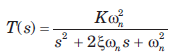has a frequency response plot shown in fig.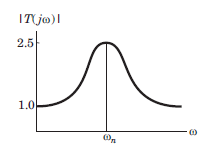Q. The system gain K is

Solution: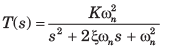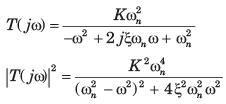From the fig. P6.5.1-2, |T(j0)| = 1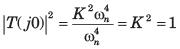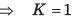QUESTION: 2

### An under damped second order system having a transfer function of the formhas a frequency response plot shown in fig.Q. The damping factor ξ is approximately

Solution:

The peak value of T(jω) occurs when the denominator of function |T(jω)|2 is minimum i.e. when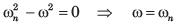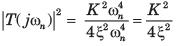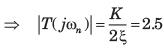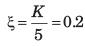QUESTION: 3

### Consider the Bode plot of a ufb system shown in fig.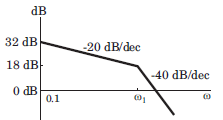Q. The steady state error corresponding to a rampinput is

Solution:

The Bode plot is as shown in fig.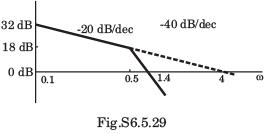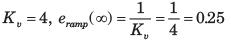QUESTION: 4

Consider the Bode plot of a ufb system shown in fig.The damping ratio is

Solution:

From Fig.: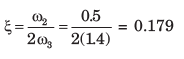QUESTION: 5

The Nyquist plot of a open-loop transfer function G(jω)H(jω) of a system encloses the (-1, j0) point. The gain margin of the system is

Solution:

If Nyquist plot encloses the point (-1, j0), the system is unstable and gain margin is negative.

QUESTION: 6

Consider a ufb system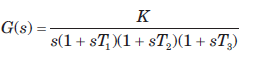The angle of asymptote, which the Nyquist plotapproaches as ω → 0 is

Solution: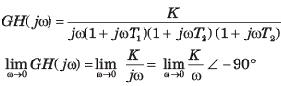Hence, the asymptote of the Nyquist plot tends to an angle of -90° as ω→ 0.

QUESTION: 7

If the gain margin of a certain feedback system isgiven as 20 dB, the Nyquist plot will cross the negativereal axis at the point

Solution: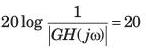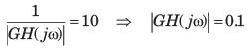Since system is stable, it will cross at s = -0.1

QUESTION: 8

The transfer function of an open-loop system is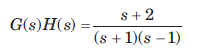The Nyquist plot will be of the form

Solution: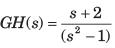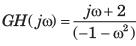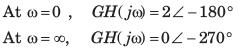Hence (B) is correct option.

QUESTION: 9

Consider a ufb system whose open-loop transfer function is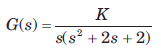The Nyquist plot for this system is

Solution: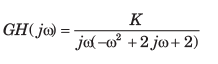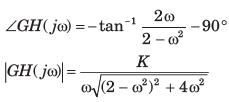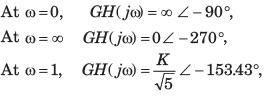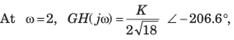Due to s there will be a infinite semicircle. Hence (C) is correct option.

QUESTION: 10

The open loop transfer function of a system is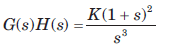The Nyquist plot for this system is

Solution: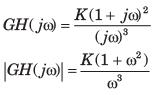∠GH(jw) = -270°+ 2 tan-1ω
For ω = 0, GH(jω) = ∞∠ -270°
For ω = 1 , ∠GH(jω) =  -180°
For ω = ∞, GH(jω) = 0 ∠ - 90°
As ω increases from 0 to ∞, phase goes -270° to -90°.
Due to s3 term there will be 3 infinite semicircle.

QUESTION: 11

For the certain unity feedback system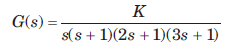The Nyquist plot is

Solution: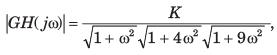∠GH(j(ω) = -90 °- tan-1 ω - tan-1 2ω - tan -1 3ω,
For ω = 0, GH(jω) = ∞∠ - 90°,
For ω = ∞, GH(jω) = 0∠- 360°,
Hence (A) is correct option.

QUESTION: 12

The Nyquist plot of a system is shown in fig. The open-loop transfer function is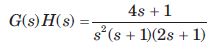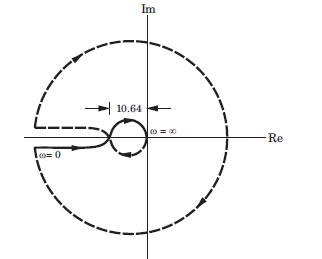The no. of poles of closed loop system in RHP are

Solution:

The open-loop poles in RHP are P = 0. Nyquist path enclosed 2 times the point (-1 + j0). Taking clockwise encirclements as negative N = -2.
N = P - Z, -2 = 0 -Z , Z = 2 which implies that two poles of closed-loop system are on RHP.

QUESTION: 13

The open-loop transfer function of a feedback control system is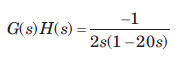Q. The Nyquist plot for this system is

Solution: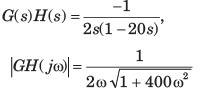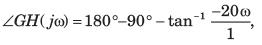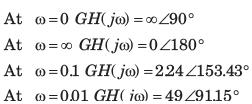QUESTION: 14

If the damping of the system becomes equal to zero, which condition of the resonant frequency is likely to occur?

Solution:
QUESTION: 15

A unity feedback system has the open loop transfer function G(s)=1/((s−1)(s+2)(s+3))
The Nyquist plot of GG encircle the origin

Solution:
QUESTION: 16

The open-loop transfer function of a feedback system is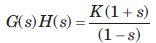Q. The Nyquist plot of this system is

Solution: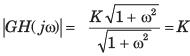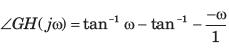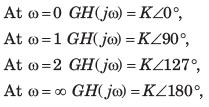QUESTION: 17

The open-loop transfer function of a feedback system isThe system is stable for K

Solution:

RHP poles of open-loop system P = 1, Z = P - N .
For closed loop system to be stable, Z = 0, 0 =1 -  N ⇒N = 1
There must be one anticlockwise rotation of point (-1+ j0). It is possible when K > 1.

QUESTION: 18

A unity feedback system has open-loop transfer function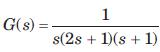Q. The Nyquist plot for the system is

Solution: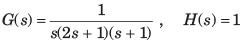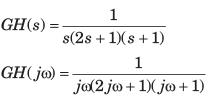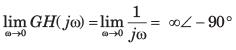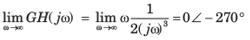The intersection with the real axis can be calculated as {GH(jω)} = 0, The condition gives ω(2ω2 -1) = 0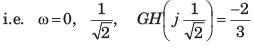With the above information the plot in option (C) is correct.

QUESTION: 19

A unity feedback system has open-loop transfer function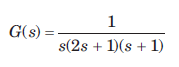Q. The phase crossover and gain crossover frequenciesare

Solution:

The Nyquist plot crosses the negative real axis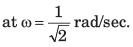Hence phase crossover frequency is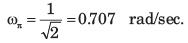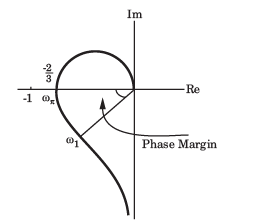The frequency at which magnitude unity is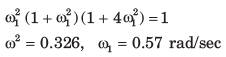QUESTION: 20

A unity feedback system has open-loop transfer functionThe gain margin and phase margin are

Solution: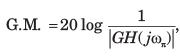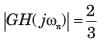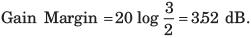∠GH(jω) = -90°- tan-1 ω- tan-1 2ω ,
At unit gain ω1 = 0.57 rad/sec,
Phase at this frequency is ∠GH(jω1) = -90°- tan-1 0.57 -tan-1 2(0.57) = -168.42°
Phase margin = -168.420+180° = 11.6°
Note that system is stable. So gain margin and phase margin are positive value. Hence only possible option is (D).